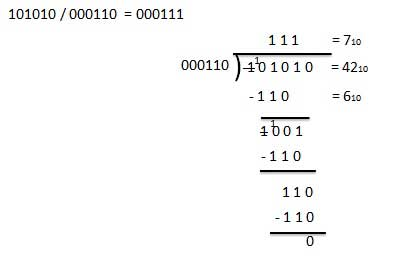# Binary Arithmetic

Binary arithmetic is essential part of all the digital computers and many other digital system.

It is a key for binary subtraction, multiplication, division. There are four rules of binary addition.In fourth case, a binary addition is creating a sum of (1 + 1 = 10) i.e. 0 is written in the given column and a carry of 1 over to the next column.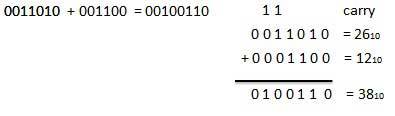## Binary Subtraction

Subtraction and Borrow, these two words will be used very frequently for the binary subtraction. There are four rules of binary subtraction.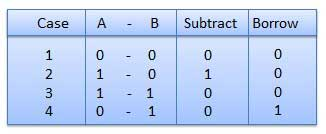### Example − Subtraction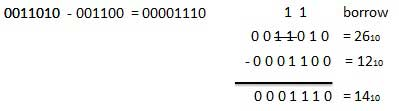## Binary Multiplication

Binary multiplication is similar to decimal multiplication. It is simpler than decimal multiplication because only 0s and 1s are involved. There are four rules of binary multiplication.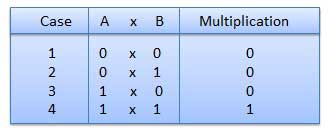### Example − Multiplication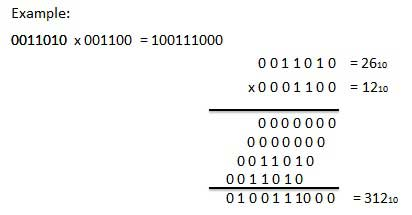## Binary Division

Binary division is similar to decimal division. It is called as the long division procedure.

### Example − Division